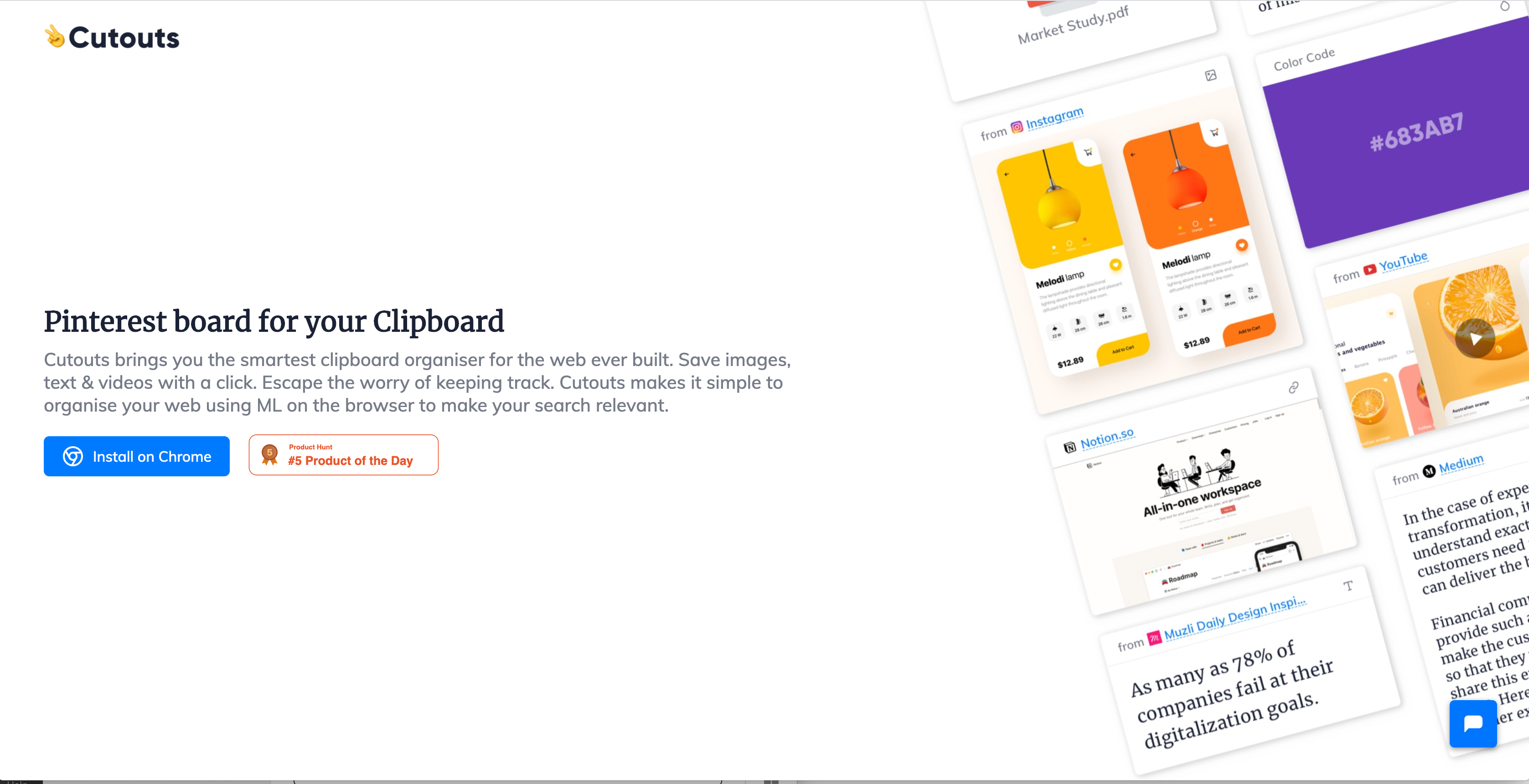# ML on Browser : Machine Learning at Cutoutscheckout https://cutouts.app/

Two weeks earlier, we launched Cutouts on producthunt ( We were in product hunt’s top 5 product of the day! Check us out here.) We got a lot of requests from people to write about building a ML model for browser- so here we are!

We wanted designed cutouts to be privacy-first, but also give users an amazing user experience. Tagging and Searching had to be taken care using Machine Learning, and the given all the constraint, it was clear to us we had to run the ML models on the browser. In comes, Tensorflow.js, which let’s you use ML directly in the browser. But Tensorflow js is still very new, so it limit what we can do using it.

# The Wrong Way

Due to my limited knowledge of the TF-js environment, my initial hypothesis was we could write any model in normal python and convert to tf-js using tensorflow js converter, and the only constraint was that the model size has to be small to be able to run in the browser. I used Keras hub to load a tiny 20 dimension Language Embedding model, and attached a dense layer to it. This was the code for creating this model-

`model = "https://tfhub.dev/google/tf2-preview/gnews-swivel-20dim/1"embedding_layer = hub.KerasLayer(model, output_shape=, input_shape=[], dtype=tf.string, trainable=False)def create_model():  model = tf.keras.Sequential([    #tf.keras.layers.Input(shape=[], dtype=tf.string),    embedding_layer,    tf.keras.layers.Dense(64, activation="relu"),    tf.keras.layers.Dense(16, activation="relu"),    tf.keras.layers.Dense(4,activation='softmax'),  ])  model.compile(loss=tf.losses.CategoricalCrossentropy(from_logits=True),      optimizer="adam", metrics=['accuracy'])  return modelmodel = create_model()`

And then I tried to convert this to a JS based model using the tensorflow converter.

After hours beating my head around the bush, I finally gave up. Tensorflow js doesn’t have support for keras hub as of now (and also a lot of custom layers that people have implemented on the internet).

Now what?

# How we got it working

We realised that we need a LM model that was available on both TFjs repository and as python implementation. We found a lite implementation for Universal Sentence Encoder was available for Tfjs as well as python.

The next step was to create embeddings vectors for the text data using the USE lite implementation in python. I used the implementation as provided by tensorflow USE docs (I ran them in batches for quicker results)

`all_embeddings = []for i in range(0,len(text_data),256):    print(i)    messages = text_data[i:i+256]        values, indices, dense_shape = process_to_IDs_in_sparse_format(sp, messages)    with tf.Session() as session:      session.run([tf.global_variables_initializer(),           tf.tables_initializer()])      message_embeddings = session.run(          encodings,          feed_dict={input_placeholder.values: values,                    input_placeholder.indices: indices,                    input_placeholder.dense_shape: dense_shape})    all_embeddings.append(np.array(message_embeddings))`

Quick Note- The python USE lite implementation is in tf version 1.15 and not the latest one. Make sure to use -

`import tensorflow.compat.v1 as tftf.disable_v2_behavior()`

. The embedding vector is of the shape (1,512) . We then trained a small CNN on the text embeddings itself.

`model = tf.keras.Sequential()model.add(tf.keras.layers.Conv1D(32,                 kernel_size=3,                 activation='tanh',                 strides=1,input_shape=(512,1)))model.add(tf.keras.layers.Flatten())# We add a vanilla hidden layer:model.add(tf.keras.layers.Dense(16,activation='relu'))model.add(tf.keras.layers.Dense(5, activation='softmax'))model.compile(loss=tf.losses.SparseCategoricalCrossentropy(from_logits=True),      optimizer="adam", metrics=['accuracy']`

And then, you can just use the tensorflow converter to actually save just this CNN model using -

`tf.keras.models.save_model(    model, 'saved_models/text_classifier_cnn/', overwrite=True, include_optimizer=True, save_format='tf',    signatures=None, options=None)`

And that’s it. Your JS model is ready.

# Browser Side Implementation

The Browser side required the two models to be downloaded, the LM model converting text to embeddings and the second model predicting the class, stitched together. The first time a cutout is saved. After that, the model is cached.

I hope this will inspire more people to build ML models for browser and be privacy first.

Next up- Building on browser models for search, Learning to rank and Image/Video Tagging. Stay Tuned!

Try out our app and help us with feedbacks! Cutouts is free and secure — https://cutouts.app/

## More from Aashay Sachdeva

Connecting the Dots.